#### Excel Functions for Beginners: A Guide to Getting Started

Excel functions are pre-built formulas that perform specific calculations. They can be used to perform complex calculations quickly and easily.

To enter a function, first select the cell where you want the result of the calculation to appear.

## Insert function

To insert a function in Excel, click the Insert Function icon.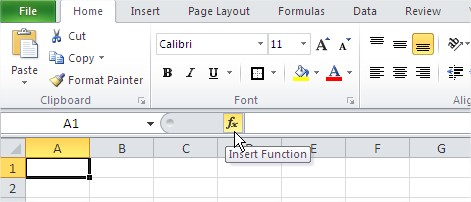In the Search function, type the name of the function or a word that describes what the function does. When you click on the name of a function, a description of the function will appear. This description can help you understand what the function does.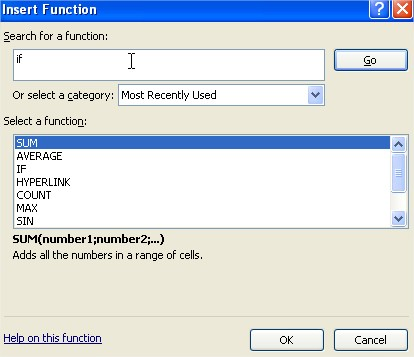## Functions categories

You can also choose the category you can find us an captivating feature, for example Financial functions.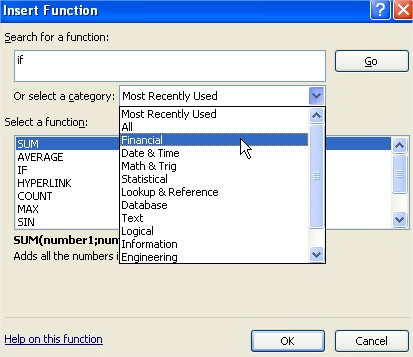Clicking on the name of the function in the window Select a function under the list of names we see a description of the function. In many cases, this description allows us to understand, for what that function is.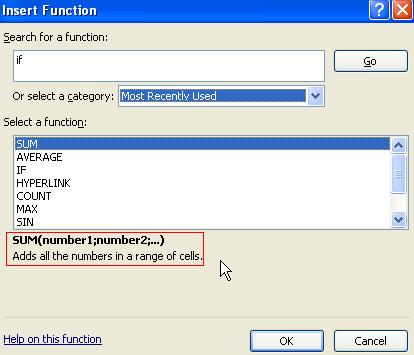The Function Arguments dialog box will appear after you select a function.

In the first pane, type the cell addresses that you want to include in the function. Below the cell addresses, you will see the current result of the function. If the function has a description, it will be displayed below the result. The description will also explain what the function does with each argument.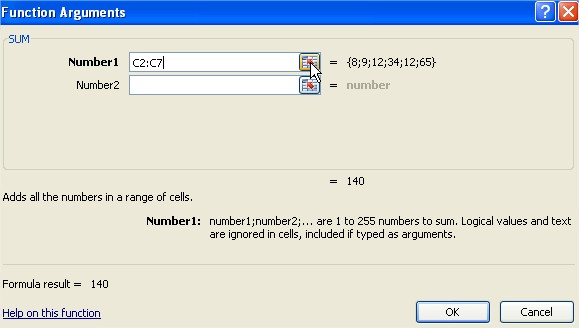There are basic information, which you must know. More you will find in this webpage.

There are many functions available in Excel, including mathematical functions, financial functions, date and time functions, and text functions. You can use functions to perform complex calculations and make your work in Excel more efficient.

There are many functions available in Excel, including mathematical functions, financial functions, date and time functions, and text functions. You can use functions to perform complex calculations and make your work in Excel more efficient.

Here are some examples of functions:

• `SUM`: This function adds the values in a range of cells.
• `AVERAGE`: This function averages the values in a range of cells.
• `COUNT`: This function counts the number of cells that contain numbers in a range of cells.
• `IF`: This function allows you to perform different calculations based on whether a condition is met.
• `VLOOKUP`: This function looks up a value in a table and returns the corresponding value from another column in the table.

## Things to Remember

• Functions are pre-built formulas that perform specific calculations.
• To insert a function, click the `Insert Function` icon.
• In the `Search` function, type the name of the function or a word that describes what the function does.
• The `Function Arguments` dialog box will appear after you select a function.
• In the first pane, type the cell addresses that you want to include in the function.
• Below the cell addresses, you will see the current result of the function.
• If the function has a description, it will be displayed below the result. The description will also explain what the function does with each argument.